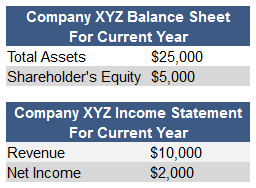## What is DuPont Identity?

The DuPont identity breaks down return on equity (ROE) into its components -- profit margin, total asset turnover, and financial leverage -- so that each one can be examined in depth.

## How Does DuPont Identity Work?

The DuPont identity is also referred to as DuPont analysis.

Before we use the DuPont identity, the basic formula for ROE is:

ROE = Profit Margin x Asset Turnover x Leverage factor

The Dupont identity breaks ROE down further:

ROE = (Net Income/Revenues) x (Revenues/Total Assets) x (Total Assets/ Shareholders' Equity)

For example, let's consider the following information for Company XYZ:Using the formula above, we can calculate that Company XYZ's ROE is:

ROE = (\$2,000/\$10,000) x (\$10,000/\$25,000) x (\$25,000/\$5,000) = 0.20 x 0.40 x 5 = 0.40 or 40%

The DuPont identity analyzes the numbers shown in profit margin (\$2,000/\$10,000), asset turnover (\$10,000/\$25,000) and leverage factor (\$25,000/\$5,000) to find Company XYZ's ROE.

## Why Does DuPont Identity Matter?

The DuPont Identity is important because it helps an analyst understand what is driving a company's ROE; profit margin is a reflection of operating efficiency; asset turnover is a reflection of the efficient use of assets; and leverage shows how much a firm relies on debt to drive profitability.

The method recognizes that in addition to profitability, it is also important to understand how efficiently a company's assets generate sales or cash and how well a company uses debt to produce incremental returns.

Using these three factors, a DuPont identity allows analysts to dissect a company, efficiently determine where the company is weak and strong and quickly know what areas of the business to look at (i.e., inventory management, debt structure, margins) for more answers. The measure is still broad, however, and is not a substitute for detailed analysis.

It is important to note that DuPont identities use both balance sheet measures (which are calculated at a fixed point in time) and income statement measures (which encompass an interval of time). As a result, major asset purchases, acquisitions, or other significant changes can distort the ROE calculation. Many analysts use average assets and shareholders' equity to mitigate this distortion, although that approach assumes the balance sheet changes occurred steadily over the course of the year, which may not be accurate either.